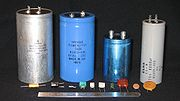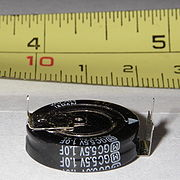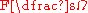xEncyclopediaSi
Si, si, or SI may refer to :- Measurement, mathematics and science :* International System of Units , the modern international standard version of the metric system...

unit of capacitance
Capacitance
In electromagnetism and electronics, capacitance is the ability of a capacitor to store energy in an electric field. Capacitance is also a measure of the amount of electric potential energy stored for a given electric potential. A common form of energy storage device is a parallel-plate capacitor...

. The unit is named after the English physicist Michael Faraday
Michael Faraday, FRS was an English chemist and physicist who contributed to the fields of electromagnetism and electrochemistry....

.

## Definition

Electric charge
Electric charge is a physical property of matter that causes it to experience a force when near other electrically charged matter. Electric charge comes in two types, called positive and negative. Two positively charged substances, or objects, experience a mutual repulsive force, as do two...

in coulombs which a capacitor
Capacitor
A capacitor is a passive two-terminal electrical component used to store energy in an electric field. The forms of practical capacitors vary widely, but all contain at least two electrical conductors separated by a dielectric ; for example, one common construction consists of metal foils separated...

will accept for the potential
Voltage
Voltage, otherwise known as electrical potential difference or electric tension is the difference in electric potential between two points — or the difference in electric potential energy per unit charge between two points...

across it to change 1 volt
Volt
The volt is the SI derived unit for electric potential, electric potential difference, and electromotive force. The volt is named in honor of the Italian physicist Alessandro Volta , who invented the voltaic pile, possibly the first chemical battery.- Definition :A single volt is defined as the...

. A coulomb is 1 ampere
Ampere
The ampere , often shortened to amp, is the SI unit of electric current and is one of the seven SI base units. It is named after André-Marie Ampère , French mathematician and physicist, considered the father of electrodynamics...

second
Second
The second is a unit of measurement of time, and is the International System of Units base unit of time. It may be measured using a clock....

. Example: A capacitor with capacitance of 47 nF will increase by 1 volt per second with a 47 mA input current.

1 microfarad (μF) = one millionth (10-6) of a farad, or 1,000,000 pF, or 1,000 nF;
1 nanofarad (nF) = one billionth (10-9) of a farad, or 1,000 pF;

### Equalities

A farad has the base SI representation of:
s
Second
The second is a unit of measurement of time, and is the International System of Units base unit of time. It may be measured using a clock....

4 × A
Ampere
The ampere , often shortened to amp, is the SI unit of electric current and is one of the seven SI base units. It is named after André-Marie Ampère , French mathematician and physicist, considered the father of electrodynamics...

2 × m-2 × kg
Kilogram
The kilogram or kilogramme , also known as the kilo, is the base unit of mass in the International System of Units and is defined as being equal to the mass of the International Prototype Kilogram , which is almost exactly equal to the mass of one liter of water...

-1

It can further be expressed as:A=ampere
Ampere
The ampere , often shortened to amp, is the SI unit of electric current and is one of the seven SI base units. It is named after André-Marie Ampère , French mathematician and physicist, considered the father of electrodynamics...

, V=volt
Volt
The volt is the SI derived unit for electric potential, electric potential difference, and electromotive force. The volt is named in honor of the Italian physicist Alessandro Volta , who invented the voltaic pile, possibly the first chemical battery.- Definition :A single volt is defined as the...

, C=coulomb, J=joule
Joule
The joule ; symbol J) is a derived unit of energy or work in the International System of Units. It is equal to the energy expended in applying a force of one newton through a distance of one metre , or in passing an electric current of one ampere through a resistance of one ohm for one second...

, m=meter, N=newton, s=second
Second
The second is a unit of measurement of time, and is the International System of Units base unit of time. It may be measured using a clock....

, W=watt
Watt
The watt is a derived unit of power in the International System of Units , named after the Scottish engineer James Watt . The unit, defined as one joule per second, measures the rate of energy conversion.-Definition:...

, kg=kilogram
Kilogram
The kilogram or kilogramme , also known as the kilo, is the base unit of mass in the International System of Units and is defined as being equal to the mass of the International Prototype Kilogram , which is almost exactly equal to the mass of one liter of water...

, Ω=ohm
Ohm
The ohm is the SI unit of electrical resistance, named after German physicist Georg Simon Ohm.- Definition :The ohm is defined as a resistance between two points of a conductor when a constant potential difference of 1 volt, applied to these points, produces in the conductor a current of 1 ampere,...

For electronics, one farad is a fairly large amount of capacitance. The most commonly used submultiples
SI prefix
The International System of Units specifies a set of unit prefixes known as SI prefixes or metric prefixes. An SI prefix is a name that precedes a basic unit of measure to indicate a decadic multiple or fraction of the unit. Each prefix has a unique symbol that is prepended to the unit symbol...

## History

The farad was coined by Josiah Latimer Clark in the year of 1861, in honor of Michael Faraday
Michael Faraday, FRS was an English chemist and physicist who contributed to the fields of electromagnetism and electrochemistry....

, but it was for a unit of quantity of charge.

## Explanation

The size of commercially available capacitors ranges from around 100 fF (femtofarads, 10-15 F) to 5 kF (kilofarads, 103 F) ultracapacitors.

When speaking of capacitor values a picofarad is sometimes referred to as a "puff" or "pic", as in "a ten puff capacitor". If the Greek letter μ
Mu (letter)
Carlos Alberto Vives Restrepo is a Grammy Award and three-time Latin Grammy Award winning-Colombian singer, composer and actor.-Biography:...

is not available, the notation uF is often used as a substitute for μF in electronics literature. A micro-microfarad (μμF), an obsolete unit sometimes found in older texts, is the equivalent of a picofarad. The millifarad is less used in practice, so that a capacitance of 4.7 F, for example, is sometimes written as 4,700 µF. North American usage also avoids nanofarads: a capacitance of 1 F will frequently be indicated as 1000 pF; and a capacitance of 1 F as 0.1 μF.

The 'farad' should not be confused with the faraday, which is the electric charge carried by one mole
Mole (unit)
The mole is a unit of measurement used in chemistry to express amounts of a chemical substance, defined as an amount of a substance that contains as many elementary entities as there are atoms in 12 grams of pure carbon-12 , the isotope of carbon with atomic weight 12. This corresponds to a value...

of singly charged ion
Ion
An ion is an atom or molecule in which the total number of electrons is not equal to the total number of protons, giving it a net positive or negative electrical charge. The name was given by physicist Michael Faraday for the substances that allow a current to pass between electrodes in a...

s.

The reciprocal of capacitance is called electrical elastance, the (non-standard, non-SI) unit of which is the daraf
Daraf
The daraf is the unit of electrical elastance , the voltage across a capacitor after accepting an electric charge of 1 coulomb; it is the reciprocal of the farad...

.

A capacitor consists of two conducting surfaces, frequently referred to as plates, separated by an insulating layer usually referred to as a dielectric
Dielectric
A dielectric is an electrical insulator that can be polarized by an applied electric field. When a dielectric is placed in an electric field, electric charges do not flow through the material, as in a conductor, but only slightly shift from their average equilibrium positions causing dielectric...

. The original capacitor was the Leyden jar
Leyden jar
A Leyden jar, or Leiden jar, is a device that "stores" static electricity between two electrodes on the inside and outside of a jar. It was invented independently by German cleric Ewald Georg von Kleist on 11 October 1745 and by Dutch scientist Pieter van Musschenbroek of Leiden in 1745–1746. The...

developed in the 18th century. It is the accumulation
Accumulation
Accumulation may refer to:* Accumulation: None, a 2002 lo-fi album* Capital accumulation, the gathering of objects of value* Glacier ice accumulation, an element in the glacier mass balance formula...

of electric charge on the plates that results in capacitance
Capacitance
In electromagnetism and electronics, capacitance is the ability of a capacitor to store energy in an electric field. Capacitance is also a measure of the amount of electric potential energy stored for a given electric potential. A common form of energy storage device is a parallel-plate capacitor...

. Modern capacitors are constructed using a range of manufacturing techniques and materials to provide the extraordinarily wide range of capacitance values used in electronics
Electronics
Electronics is the branch of science, engineering and technology that deals with electrical circuits involving active electrical components such as vacuum tubes, transistors, diodes and integrated circuits, and associated passive interconnection technologies...

applications from femtofarads to farads, with voltage withstand capabilities ranging from a few volt
Volt
The volt is the SI derived unit for electric potential, electric potential difference, and electromotive force. The volt is named in honor of the Italian physicist Alessandro Volta , who invented the voltaic pile, possibly the first chemical battery.- Definition :A single volt is defined as the...

s to several kilovolts.

One picofarad is about the smallest value of capacitor available for general use in electronic design, since smaller capacitors would be dominated by the parasitic capacitances (stray capacitance) of other components, wiring or printed circuit board
Printed circuit board
A printed circuit board, or PCB, is used to mechanically support and electrically connect electronic components using conductive pathways, tracks or signal traces etched from copper sheets laminated onto a non-conductive substrate. It is also referred to as printed wiring board or etched wiring...

s. When capacitance values of 1 pF or lower are required, engineers sometimes create their own capacitors by twisting two short lengths of insulated wire together.

The capacitance of the Earth's ionosphere
Ionosphere
The ionosphere is a part of the upper atmosphere, comprising portions of the mesosphere, thermosphere and exosphere, distinguished because it is ionized by solar radiation. It plays an important part in atmospheric electricity and forms the inner edge of the magnetosphere...

with respect to the ground is calculated to be about 1.8 F.

An abfarad is an obsolete electromagnetic (CGS) unit of capacitance equal to 109 farads (1,000,000,000 F or 1 GF
Giga
Giga is a unit prefix in the metric system denoting a factor of billion . It has the symbol G.Giga is derived from the Greek γίγας, meaning 'giant'...

). This very large unit is used in medical terminology only.

The statfarad was the comparable obsolete electrostatic (CGS) unit of capacitance, and was equal to 1 microfarad.